Courses

# NCERT Solutions,Thrust Area and Pressure, Class 9, Science Class 9 Notes | EduRev

## Class 9 : NCERT Solutions,Thrust Area and Pressure, Class 9, Science Class 9 Notes | EduRev

The document NCERT Solutions,Thrust Area and Pressure, Class 9, Science Class 9 Notes | EduRev is a part of Class 9 category.
All you need of Class 9 at this link: Class 9

NCERT Solutions,Thrust Area and Pressure, Class 9, Science

Ncert questions with solution

1. State the universal law of gravitation.

Ans. Every body in this universe attracts every other body with a force, which is directly proportional to the product of their masses and inversely proportional to the square of distance between their centres.

2. Write the formula to find the magnitude of the gravitational force between the earth and an object on the surface of the earth.

Ans. Fgravitation =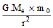Where G is gravitational constant

Me = the mass of the earth

m0 = the mass of the object

r = the distance between the centre of gravity of the object and the centre of the earth.

3. What do you mean by free fall ?

Ans. When an object falls with a constant acceleration, under the influence of force of gravitation of the earth, object is said to have free fall.

4. What do you mean by acceleration due to gravity ?

Ans. The acceleration produced in a body due to force of gravity is called acceleration due to gravity. It is denoted by g. The value of acceleration due to gravity is taken as 9.8 ms2 at the sea level.

5. What are the differences between the mass of an object and its weight ?

Ans. Mass Weight

1. It is the amount of mater in a body. 1. It is the force of gravity acting on a body.

2. It is measured by a physical balance. 2. It is measured by a spring balance.

3. It is constant at all the places in universe. 3. It is variable and changes with the change in acceleration due to gravity

4. Its S.I. unit is kilogram. 4. Its S.I. unit is newton.

6. Why is the weight of an object on the moon th its weight on the earth ?

Ans. Mass of the moon (M) = 7.4 × 1022 kg

Radius of the moon (R) = 1.74 × 106 m

Gravitational constant (G) = 6.7 × 1011 Nm2kg2

Acceleration due to gravity =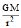on moon (gm)

gm =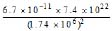Gravitation =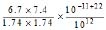gm = 1.63 ms2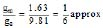gm = ge

7. You find your mass to be 42 kg on a weighing machine. Is your mass more or less than 42 kg?

Ans. Mass is always a constant quantity. Therefore, it cannot be more or less than 42 kg.

8. You have a bag of cotton and an iron bar, each indicating a mass of 100 kg when measured on a weighing machine. In reality, one is heavier than other. Can you say which one is heavier and why?

Ans. Iron heavier

Reason : Heaviness of an object is determined by its density. As the iron has far more density than cotton, therefore, in reality iron is heavier than cotton.

9. How does the force of gravitation between two objects change when the distance between them is reduced to half ?

Ans. When all other variables remain constant, the force of gravitation is inversely proportional to the square of distance between the two objects.

F µ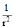Þ F µ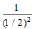= 4

the force of gravitation increases 4 times.

10. Gravitational force acts on all objects in proportion to their masses. Why then, a heavy object does not fall faster than a light object ?

Ans. A freely falling object of any mass falls under the action of gravity given by

g = where 'G' is constant to gravitation

M ® Mass of earth

r ® the distance between the object and the centre of earth.

As, the acceleration due to gravity is independent of the mass of the objects.

all object fall at the same speed towards the earth.

11. What is the magnitude of the gravitational force between the earth and a 1 kg object on its surface? (Mass of the earth is 6 × 1024 kg and radius of the earth is 6.4 × 106 m.)

Ans. Fgravitation =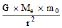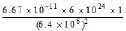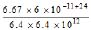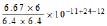= 0.9770 × 10 N = 9.770 N 9.8 N

12. The earth and the moon are attracted to each other by gravitational force. Does the earth attract the moon with a force that is greater or smaller or the same as the force with which the moon attracts the earth ? Why ?

Ans. The earth attracts the moon with the same force with which the moon attracts the earth because, the gravitational force between any two bodies is mutual and equal according to Newton's universal law of gravitation.

13. If the moon attracts the earth, why does the earth not move towards the moon ?

Ans. The earth does not move towards the moon because the force exerted by the earth or the moon on each other is insufficient to move the earth on account of its huge mass.

14. What happens to the force between two objects, if

(i) the mass of one object is doubled ?

(ii) the distance between the objects is doubled and tripled ?

(iii) the masses of both objects are doubled ?

Ans. (i) The force of gravitation doubles.

(ii) The force of gravitation decreases 4 times if the distance between the objects is doubled and if the distance between the objects is tripled then the force of gravitation decreases 9 times.

(iii) The force of gravitation increases 4 times.

15. What is the importance of universal law of gravitation ?

Ans. Importance of universal law of gravitation is as follows :

(i) It is the gravitational force between the sun and the earth, which makes the earth to move around
the sun with a uniform speed.

(ii) The tides formed in sea are because of gravitational pull exerted by the sun and the moon on the surface of water.

(iii) It is the gravitational pull of earth, which keeps us and other bodies firmly on the ground.

(iv) It is the gravitational pull of the earth, which holds our atmosphere in place.

16. What is the acceleration of free fall ?

Ans. The average acceleration of free fall on the surface of earth is 9.81 ms2.

17. What do we call the gravitational force between the earth and an object ?

Ans. It is called force of gravity.

18. Amit buys few grams of gold at the poles as per the instruction of one of his friends. He hands over the same when he meets him at the equator. Will the friend agree with the weight of gold bought ? If not, why? [Hint : The value of g is greater at the poles than at the equator]

Ans. Weight of an object = mg

where 'm' is mass of the object at the equator than at the poles as the magnitude of 'g' is less at the equator than at the poles.

So, his friend will not agree with weight of the gold at the poles when measured at equator.

19. Why will a sheet of paper fall slower than one that is crumpled into a ball ?

Ans. Sheet crumpled into a ball has small surface area as compared to the similar unfolded sheet. Therefore unfolded sheet will experience more friction due to air as compared to the sheet crumpled into a ball inspite of same force of gravity acts upon them. It is larger friction of air which slows down the unfolded sheet and therefore it falls slower as compared to sheet crumpled into a ball.

20. Gravitational force on the surface of the moon is only as strong as gravitational force on the earth. What is the weight in newton of a 10 kg object on the moon and on the earth?

Ans. Mass of object (m) = 10 kg

Acceleration due to gravity on earth (ge) = 9.81 ms2.

Acceleration due to gravity on moon (gm) = ms2.

Weight of the object on the earth = mge = 10 × 9.81 = 98.1 N

Weight of the object on the moon = mgm = 10 ×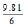= 16.35 N

21. A ball is thrown vertically upwards with a velocity of 49 ms1. Calculate

(i) The maximum height to which it rises.

(ii) The total time it takes to return to the surface of the earth.

Ans. (i) Initial velocity of the ball (u) = 49 ms1

Final velocity of the ball (v) = 0

Acceleration due to gravity (g) = 9.8 ms2

[In upward direction g is ve]

Height attained by the ball (S) = ?

Time for rising up (t) = ?

We know ; v2 u2 = 2gS

(0)2 (49)2 = 2 × (9.8) × S

S =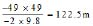We know v = u gt

0 = 49 9.8 × t

Þ t =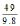Þ t = 5s

(ii) Now, time for upward journey of the ball = the time for downward journey of the ball.

Total time taken by the ball to return to the surface of earth = 2 × t = 2 × 5 = 10 s

22. A stone is released from the top of a tower of height 19.6 m. Calculate its final velocity just before touching the ground.

Ans. Initial velocity of stone (u) = 0

Final velocity of stone (v) = ?

Height attained (h) = 19.6 m

Acceleration due to gravity (g) = 9.8 ms2

We know ; v2 = u2  2gh

v2 = (0)2  2 × 9.8 × 19.6

v2 = 19.6 × 19.6

v =v = 19.6 ms1.

23. A stone is thrown vertically upward with an initial velocity of 40 ms1. Taking g = 10 ms2, find the maximum height reached by the stone. What is the net displacement and the total distance covered by the stone ?

Ans. Initial velocity of stone (u) = 40 ms1

Final velocity of stone (v) = 0

Acceleration due to gravity (g) = 10 ms2

[For upward direction g is ve]

Height attained by stone (h) = ?

We, know, v2 u2 = 2gh

(0)2 (40)2 = 2 × (10) × h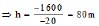Maximum height attained by stone = 80 m

Net displacement of stone = 0

(because the stone returns back to the same point)

Total distance covered by the stone = 2 × height attained = 2 × 80 = 160 m

24. Calculate the force of gravitation between the earth and the Sun, given that the mass of the earth
= 6 × 1024 kg and of the Sun = 2 × 1030 kg. The average distance between the two is 1.5 × 1011 m.

Ans. Fgravitation =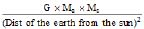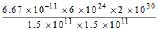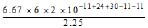= 35.57 × 1021 N

25. A stone is allowed to fall from the top of a tower 100 m high and at the same time another stone is projected vertically upwards from the ground with a velocity of 25 ms1. Calculate when and where the two stones will meet.

Ans. Acceleration due to gravity (g) = 10 ms2

Initial velocity (u) = 0

Distance

(S) = 100 x

Time (t) = ?

S = ut gt2

(100 x) = 0 × t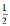× 10 t2

Þ 100 x = 5t2 ... (1)

For the stone moving vertically upwards :

Initial velocity (u) = 25 ms1

Time (t) = ?

Acceleration due to gravity (g) = 10 ms1

[In upward direction g is ve]

Distance (S) = x

we know ;

S = ut gt2

x = 25 × t × (10 t2)

Þ x = 25t 5t... (2)

Substituting the value of x from (2) in (1) we get,

100 (25t 5t2) = 5t2

100 25t 5t2 = 5t2

25 t = 100

t = 4 s

Put the value of t in (1)

(1) Þ 100 x = 5(4)2

Þ 100 x = 80

x = 20 m

the stones will meet at a height of 20 m from ground after 4 s.

26. A ball thrown up vertically returns to the thrower after 6 s. Find.

(a) the velocity with which it was thrown up.

(b) the maximum height it reaches and

(c) its position after 4 s

Ans. Q the ball returns back to the thrower in 6 s, the time for its upward journey = 6 ÷ 2 = 3s

For the upward motion of ball

Initial velocity (u) = ?

Final velocity (v) = 0 (Q Ball comes to rest)

Time (t) = 3s

Acceleration due to gravity (g) = 10ms2

[In upward direction g is ve]

We know ;

v = u gt

Þ 0 = u 10 × 3

Þ u = 30

Þ u = 30 ms1

We know ;

S = ut gt2

S = 30 × 3 × 10 × (3)2

Þ S = 90 45

S = 45 m

For the downward motion of ball

Initial velocity (u) = 0

Time for downward fall (t) = 4 3 = 1 s

Acceleration due to gravity (g) = 10 ms2

Distance covered in downward direction (S) = ?

We know ;

S = ut gt2

S = 0 × t × 10 × (1)2

Þ S = 0 5

Þ S = 5 m

Position of ball after 4 s from ground

= 45 5

= 40 m.

,

,

,

,

,

,

,

,

,

,

,

,

,

,

,

,

,

,

,

,

,

,

,

,

,

,

,

,

,

,

,

,

,

,

,

;